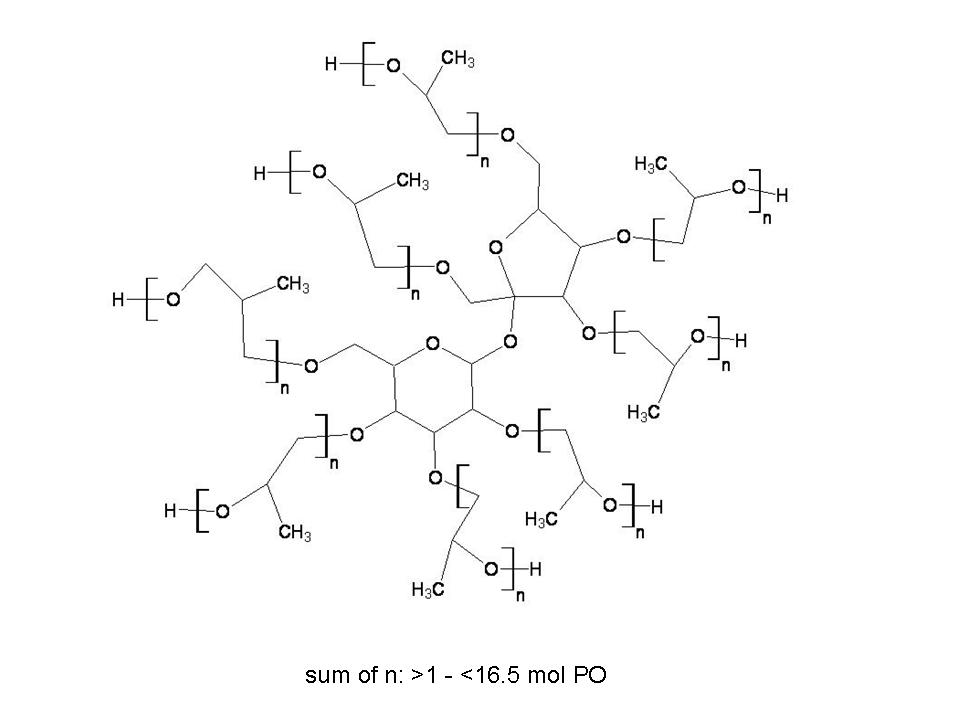## Diss Factsheets## Substance identity

### IdentificationDisplay Name:
Sucrose, propoxylated
EC Number:
500-029-3
EC Name:
Sucrose, propoxylated
CAS Number:
9049-71-2
Molecular formula:
C12H14O11-(C3H6O)nH8 sum of n: >1 - <16.5 mol PO
IUPAC Name:
Sucrose, propoxylated

Composition:
UVCB
Origin:
organic

### Substance Identifiers

• Petol PZ 360-4G
• Petol PZ 380-4G
• Petol PZ 400-4G
• Petol PZ 400-5G
• Petol PZ 440-4T
• Petol PZ 480 4G
• Petol PZ 480-4T
• Voranol* 370 Polyol
• Voranol* 370 Polyol
• ### Compositions

#### Boundary Composition(s)

State Form:
liquid

##### Constituent 1Reference substance name:
Sucrose
EC Number:
200-334-9
EC Name:
Sucrose
CAS Number:
57-50-1
Molecular formula:
C12H22O11
IUPAC Name:
(2R,3R,4S,5R,6R)-2-[(2S,3S,4R,5R)-3,4-Dihydroxy-2,5-bis(hydroxymethyl)tetrahydrofur-2-yloxy]-6-(hydroxymethyl)tetrahydro-2H-pyran-3,4,5-triol
##### Constituent 2Reference substance name:
Reaction product of 1 eq. of Sucrose with 1 eq. of Propylene oxide (x=1)
Molecular formula:
C15H28O12
IUPAC Name:
Reaction product of 1 eq. of Sucrose with 1 eq. of Propylene oxide (x=1)
##### Constituent 3Reference substance name:
Reaction product of 1 eq. of Sucrose with 2 eq. of Propylene oxide (x=2)
Molecular formula:
C18H34O13
IUPAC Name:
Reaction product of 1 eq. of Sucrose with 2 eq. of Propylene oxide (x=2)
##### Constituent 4Reference substance name:
Reaction product of 1 eq. of Sucrose with 3 eq. of Propylene oxide (x=3) RG136
Molecular formula:
C21H40O14
IUPAC Name:
Reaction product of 1 eq. of Sucrose with 3 eq. of Propylene oxide (x=3) RG136
##### Constituent 5Reference substance name:
Reaction product of 1 eq. of Sucrose with 4 eq. of Propylene oxide (x=4)
Molecular formula:
C24H46O15
IUPAC Name:
Reaction product of 1 eq. of Sucrose with 4 eq. of Propylene oxide (x=4)
##### Constituent 6Reference substance name:
Reaction product of 1 eq. of Sucrose with 5 eq. of Propylene oxide (x=5)
Molecular formula:
C27H52O16
IUPAC Name:
Reaction product of 1 eq. of Sucrose with 5 eq. of Propylene oxide (x=5)
##### Constituent 7Reference substance name:
Reaction product of 1 eq. of Sucrose with 6 eq. of Propylene oxide (x=6)
Molecular formula:
C30H58O17
IUPAC Name:
Reaction product of 1 eq. of Sucrose with 6 eq. of Propylene oxide (x=6)
##### Constituent 8Reference substance name:
Reaction product of 1 eq. of Sucrose with 7 eq. of Propylene oxide (x=7)
Molecular formula:
C33H64O18
IUPAC Name:
Reaction product of 1 eq. of Sucrose with 7 eq. of Propylene oxide (x=7)
##### Constituent 9Reference substance name:
Reaction product of 1 eq. of Sucrose with 8 eq. of Propylene oxide (x=8)
Molecular formula:
C36H70O19
IUPAC Name:
Reaction product of 1 eq. of Sucrose with 8 eq. of Propylene oxide (x=8)
##### Constituent 10Reference substance name:
Reaction product of 1 eq. of Sucrose with 9 eq. of Propylene oxide (x=9)
Molecular formula:
C39H76O20
IUPAC Name:
Reaction product of 1 eq. of Sucrose with 9 eq. of Propylene oxide (x=9)
##### Constituent 11Reference substance name:
Reaction product of 1 eq. of Sucrose with 10 eq. of Propylene oxide (x=10)
Molecular formula:
C42H82O21
IUPAC Name:
Reaction product of 1 eq. of Sucrose with 10 eq. of Propylene oxide (x=10)
##### Constituent 12Reference substance name:
Reaction product of 1 eq. of Sucrose with 11 eq. of Propylene oxide (x=11)
Molecular formula:
C45H88O22
IUPAC Name:
Reaction product of 1 eq. of Sucrose with 11 eq. of Propylene oxide (x=11)
##### Constituent 13Reference substance name:
Reaction product of 1 eq. of Sucrose with 12 eq. of Propylene oxide (x=12)
Molecular formula:
C48H94O23
IUPAC Name:
Reaction product of 1 eq. of Sucrose with 12 eq. of Propylene oxide (x=12)
##### Constituent 14Reference substance name:
Reaction product of 1 eq. of Sucrose with 13 eq. of Propylene oxide (x=13)
Molecular formula:
C51H100O24
IUPAC Name:
Reaction product of 1 eq. of Sucrose with 13 eq. of Propylene oxide (x=13)
##### Constituent 15Reference substance name:
Reaction product of 1 eq. of Sucrose with 14 eq. of Propylene oxide (x=14)
Molecular formula:
C54H106O25
IUPAC Name:
Reaction product of 1 eq. of Sucrose with 14 eq. of Propylene oxide (x=14)
##### Constituent 16Reference substance name:
Reaction product of 1 eq. of Sucrose with 15 eq. of Propylene oxide (x=15)
Molecular formula:
C57H112O26
IUPAC Name:
Reaction product of 1 eq. of Sucrose with 15 eq. of Propylene oxide (x=15)
##### Constituent 17Reference substance name:
Reaction product of 1 eq. of Sucrose with 16 eq. of Propylene oxide (x=16)
Molecular formula:
C60H118O27
IUPAC Name:
Reaction product of 1 eq. of Sucrose with 16 eq. of Propylene oxide (x=16)
##### Constituent 18Reference substance name:
Reaction product of 1 eq. of Sucrose with >=17 eq. of Propylene oxide (x>=17)
Molecular formula:
not applicable
IUPAC Name:
Reaction product of 1 eq. of Sucrose with >=17 eq. of Propylene oxide (x>=17)

#### Legal Entity Composition(s)

State Form:
liquid

##### Constituent 1Reference substance name:
Sucrose
EC Number:
200-334-9
EC Name:
Sucrose
CAS Number:
57-50-1
Molecular formula:
C12H22O11
IUPAC Name:
(2R,3R,4S,5R,6R)-2-[(2S,3S,4R,5R)-3,4-Dihydroxy-2,5-bis(hydroxymethyl)tetrahydrofur-2-yloxy]-6-(hydroxymethyl)tetrahydro-2H-pyran-3,4,5-triol
##### Constituent 2Reference substance name:
Reaction product of 1 eq. of Sucrose with 1 eq. of Propylene oxide (x=1)
Molecular formula:
C15H28O12
IUPAC Name:
Reaction product of 1 eq. of Sucrose with 1 eq. of Propylene oxide (x=1)
##### Constituent 3Reference substance name:
Reaction product of 1 eq. of Sucrose with 2 eq. of Propylene oxide (x=2)
Molecular formula:
C18H34O13
IUPAC Name:
Reaction product of 1 eq. of Sucrose with 2 eq. of Propylene oxide (x=2)
##### Constituent 4Reference substance name:
Reaction product of 1 eq. of Sucrose with 3 eq. of Propylene oxide (x=3) RG136
Molecular formula:
C21H40O14
IUPAC Name:
Reaction product of 1 eq. of Sucrose with 3 eq. of Propylene oxide (x=3) RG136